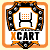View Single Post
#4
 elmirage001X-Wizard Join Date: Apr 2007 Posts: 1,858Re: Admin Category Products Sale Updater

I was using this yesterday morning and thought of a way to make it much easier and super quick. What if there was a pull down where I could select the discount and then click update and the price, list price, and On Sale Y/N selection would all be done for me. So I spent a few hours in the afternoon working on it.

Please feel free to post all edits/corrections. I've not fully tested if you don't have the "On Sale" Module. Test on your development store before using on your live store. Use at your own risk as I only know enough to be dangerousEdited: This only works for items < \$1000 !!!

The below attachment shows how it works.On the attachment you can see the new column added to set the discount amount. I had to add a field to the 'xcart_products' table called 'set_discount'.

Code:
`ALTER TABLE `xcart_products` ADD `set_discount` INT NOT NULL DEFAULT '99';`

I set the default amount to 99. A value of 99 is the manual mode and with all products having a 99 default value no changes are made to existing pricing. I only use the 'list price' when I have something on sale and it's zero the rest of the time. If you use 'list price' differently you'll have to edit the code.

The edited code sections are below and replace the original 3 edits from the above post.

Code:
```if (\$v['set_discount'] != '99') {

if (\$v['list_price'] > 0) {
\$v["on_sale"] = 'Y';
\$v['price'] = \$v['list_price'] * ((100- \$v['set_discount'])/100);
} else {
\$v["on_sale"] = 'N';
if (\$v['set_discount'] > 0) {
\$v['list_price'] = \$v['price'];
\$v['price'] = \$v['list_price'] * ((100- \$v['set_discount'])/100);
\$v["on_sale"] = 'Y';
}
}

if (\$v['set_discount'] == 0) {
\$v["on_sale"] = 'N';
if (\$v['list_price'] > 0) {
\$v['price'] = \$v['list_price'];
\$v['list_price'] = 0;
}
}
}

if (isset(\$v['list_price']) && is_numeric(\$v['list_price']))
\$update[] = "list_price='".strval(\$v["list_price"])."'";

if (isset(\$v['set_discount']) && is_numeric(\$v['set_discount']))
\$update[] = "set_discount='".strval(\$v["set_discount"])."'";

if (isset(\$v['on_sale']))
\$update[] = "on_sale='".(\$v["on_sale"])."'";```

Code:
```<td nowrap="nowrap">{\$lng.lbl_list_price} ({\$config.General.currency_symbol})</a></td>
<td nowrap="nowrap">Discount %</td>
<td nowrap="nowrap">Set Discount</td>
{if \$active_modules.On_Sale}
<td nowrap="nowrap">{\$lng.lbl_on_sale}</a></td>
{/if}```

Code:
```<td align="center">
{if \$products[prod].product_type ne 'C'}
{/if}
</td>

<td align="center">
{if \$products[prod].product_type ne 'C'}
{if \$products[prod].list_price gt 0 && \$products[prod].price gt 0}
{if \$products[prod].list_price gt \$products[prod].price}
{math equation="100 - (ourPrice / listPrice * 100)"
ourPrice=\$products[prod].price
listPrice=\$products[prod].list_price format="%.2f"}%
{elseif \$products[prod].list_price == \$products[prod].price}
0%
{else}
P>L ?
{/if}
{/if}
{/if}
</td>

<td align="center">
{if \$products[prod].product_type ne 'C'}
<select name="posted_data[{\$products[prod].productid}][set_discount]">
<option value="0"  {if \$products[prod].set_discount eq 0} selected="selected"{/if}>0</option>
<option value="10" {if \$products[prod].set_discount eq 10} selected="selected"{/if}>10</option>
<option value="15" {if \$products[prod].set_discount eq 15} selected="selected"{/if}>15</option>
<option value="20" {if \$products[prod].set_discount eq 20} selected="selected"{/if}>20</option>
<option value="99" {if \$products[prod].set_discount eq 99} selected="selected"{/if}>99</option>
</select>
{/if}
</td>

{if \$active_modules.On_Sale}
<td align="center">
{if \$products[prod].product_type ne 'C'}
<select name="posted_data[{\$products[prod].productid}][on_sale]">
<option value="N" {if \$products[prod].on_sale eq "N"} selected="selected"{/if}>{\$lng.lbl_no}</option>
<option value="Y" {if \$products[prod].on_sale eq "Y"} selected="selected"{/if}>{\$lng.lbl_yes}</option>
</select>
{/if}
</td>
{/if}  ```
__________________
X-Cart GoldPlus v4.7.12 | reBOOT (reDUX) Template | Live
X-Cart GoldPlus v4.7.12 | reBOOT (reDUX) Template | Dev
• XCARTMODS.CO.UK | reBOOT (reDUX) |
• Performive Hosting | Linux | PHP v7.4.15 | MySQL v5.7.33 |